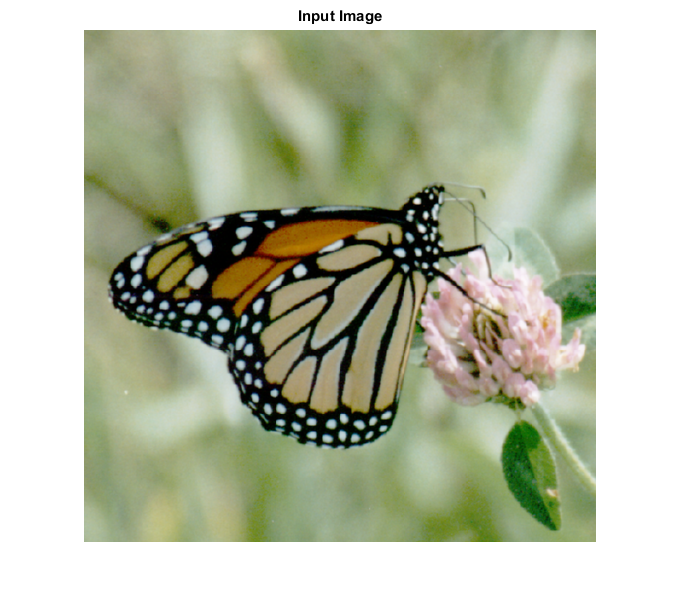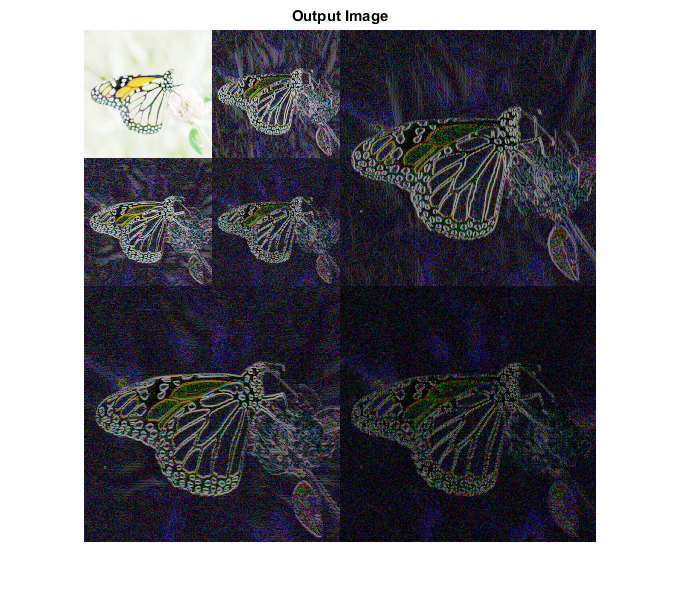# WAVHAAR_CVIP

wavhaar_cvip() - performs forward wavelet transform based on Haar wavelet

## SYNTAX

`[ out_mat ] = wavhaar_cvip( img,dec )`

Input Parameters Include :

• img - The orignial image which can be grayscale or RGB.
• dec - The decomposition level. An integer greater than or equal to 1.

Output Parameter Include :

• out_mat - The wavelet transform of the image using Haar wavelet coefficients.

## DESCRIPTION

The function performs the forward wavelet transform on an input image based on the Haar transform. The Haar transform has rectangular waves as the basis functions. As the decomposition level dec, given as input by the user increases, the resolution in the Haar transform increases and as a result edge information increases. The decomposition is applied on the lowpass-lowpass verision of the image.

## REFERENCE

1. Scott E Umbaugh. DIGITAL IMAGE PROCESSING AND ANALYSIS: Applications with MATLAB and CVIPtools, 3rd Edition.

## EXAMPLE

```% Read image

b = double(a);

% Decomposition level

dec = 2;

% call function

w = wavhaar_cvip(b,dec);

% This is a good way to remap

S = log(1+abs(w));

S = remap_cvip(S);

% Display input image

figure;imshow(a);title('Input Image');

% Display output image

figure; imshow(S,[]);title('Output Image');  % tranforms for display.
```